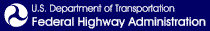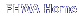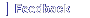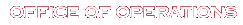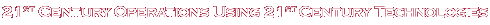# Application of Travel Time Data and Statistics to Travel Time Reliability Analyses: Handbook and Support Materials

## Chapter 3. Data Processing for Reliability

### Setting Spatial and Temporal Scales

The data available for measuring travel time reliability is of high resolution and it is useful to aggregate the data spatially and temporally. For performance measurement and project planning, the two most reasonable spatial scales are the facility and the trip. The Highway Capacity Manual (HCM) defines a facility as: “a length of roadway, bicycle path, or pedestrian walkway composed of a connected series of points and segments.” A reasonable length for a facility can be determined as the roadway segments between major intersections or interchanges. In urban areas, these distances can be 3–7 miles in length. Additionally, trips can be defined between origin and destination points of interest. A trip can take a consistent path of connected roadways, or they can take any roadway path between origins and destinations.

The analyst also defines the periods to be analyzed. At a minimum, the morning and afternoon peak periods for nonholiday weekdays are a good practice used since these are the times when congestion will be most apparent. The beginning and ending times of the peak periods are based on local knowledge of traffic patterns. The ending time should be late enough to capture residual queue dispersion from the peak.

### Travel Time Calculation Methods

Given the differences in the various data sources, four travel time calculation methods were explored:

• Detector
• Probe snapshot
• Probe virtual
• Trajectory

For detector data (“spot” speeds and volumes), travel times are synthesized for the lowest level of aggregation present in the data. The assumption is that the spot speeds are uniform across a length of highway equal to the half the distance to the nearest upstream and downstream detectors. Detector spacing significantly affects the accuracy of this assumption—the closer the spacing, the more reasonable the assumption. The steps in this aggregation process are shown in figure 4 and are as follows.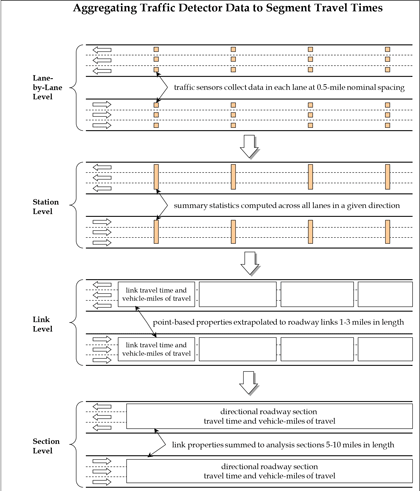Source: FHWA.

Figure 4. Flow chart. Creating segment (facility) travel times.19

How lane-level roadway detector measurements of speed and volume are aggregated to produce section level travel times. The levels of aggregation are: 1) sum across all lanes; 2) compute travel times between detectors; and 3) sum all travel times across all detectors on a section.

#### Step 1: Combine Lane Data into Station Data

If data are reported by lane, the lane-by-lane data are combined into a station (e.g., all lanes in a direction). Traffic volumes are summed across all lanes, and the traffic speed is reported as a weighted average, with weighting based on the respective lane traffic volumes. If volume data are missing for any of the lanes, the total station volume is factored up by the ratio of the total number of lanes to the number of lanes with valid data.

#### Step 2: Calculate Link Statistics

Link properties are estimated from station data by assuming that each station has a zone of influence equal to half the distance to the detectors immediately upstream and downstream (the detector zone length). The measured speeds are then assumed to be constant within each zone of influence.

• Vehicle-miles traveled (VMT) is the volume times the detector zone length.
• Vehicle-hours traveled (VHT) is the VMT divided by the minimum of free-flow speed and speed.
• Travel time (in hours) is the detector zone length divided by the speed.

#### Step 3: Calculate Section Statistics

Section VMT, VHT, and travel time is the sum of these measures for each link within the section. If any data are missing for any of the stations, the total section VMT, VHT, or travel time is factored up by the ratio of the total section length to the total length of stations with valid data.

The following measures are the computed for the section:

• Space-mean speed is the VMT divided by VHT
• Travel rate is the reciprocal of space-mean speed
• Travel time is the facility length divided by the space-mean speed

The probe snapshot method uses probe data and is based on the detector method, which develops the 5-minute facility travel times by summing up all section travel times along the facility at each given time interval. The facility travel times can be adjusted based on the ratio of sum of the section lengths and facility length if missing section data exists. Facility-level space-mean speeds can be derived from the facility travel times.

The probe virtual method relies on an algorithm that synthesizes travel times by simulating vehicles on the time/space diagram developed from probe data. A vehicle’s speed at any given moment is determined by what link it is on at a given time. As it takes time for a vehicle to travel to a specific section, the traffic condition on that section could change by the time the vehicle arrives. In this way, end-to-end travel times are created and compiled into a travel time distribution from which reliability measures are calculated.

The trajectory method will be customized based on the nature of the data. For each trip that can be identified by the common trip ID from both ends of the facility, the direction of the trip is first determined based on the difference in adjacent locations. As the origin and destination ends can be defined once the direction is set, trip travel time is calculated by subtracting the earliest timestamp in the origin end from the latest timestamp in the destination end. Additionally, to account for the trips that potentially stopped or made detours, an error removal procedure was developed using mean absolute deviation (MAD) test based on travel time (figure 5):Figure 5. Equation. Formula for mean absolute deviation.

Where:

TTi: = travel time for vehicle i.
M = median of the 15-minute block travel times.
n = number of observations in the block.

To ensure the correct traffic context of the individual trip, trips are grouped into 15-minute blocks first to develop the baseline travel time (M). An error is defined as one that is out of the following range (figure 6):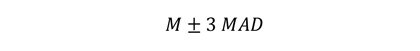Figure 6. Equation. Formula for identifying errors.

In practice, the size of the block and the number of MAD (sensitivity coefficient) can be adjusted based on the field data.

### Analysis Procedures

The analysis procedure developed in this study includes data extraction, data transformation, data aggregation and measures calculation.

#### Data Extraction

The candidate facilities were first determined based on the facility characteristics (e.g., roadway type, congestion level) and the travel time data availability along the facilities. The data extraction step uses the input of a dataset with larger geographical coverage and the facility definition, and outputs the dataset specifically for the selected facility.

For the probe and detector data, the TMCs or detector stations representing the subsections of a road can be identified once its physical extent is determined. The road dataset can be produced by subsetting the entire dataset based on the TMCs or detector stations. TMCs and detector stations are directional therefore data for each direction can be extracted separately.

As no subsection structure exists in the trajectory data, a method that utilizes the trajectory trip ID and timestamp was developed. This method starts by defining two polygons at both ends of a facility. The polygons should be as small as possible to avoid capturing trips on other facilities but big enough to fully cover the two travel directions of the facility end. Trajectory data points that fall within the polygons can be extracted separately according to their respective polygons. The common trip ID and timestamp can then be used to establish the information of the trips that traveled from one end to the other.

One polygon that covers the entire study facility is not good practice as it cannot guarantee that all trips start at one end and traveled to the other end. Trips entered or left in the middle of the facility from/to a side street also could be included in the extracted dataset, which could skew the travel time calculation. Depending on the facility geometry, one big polygon also might contain data points on adjacent streets, which could further complicate the calculation.

#### Data Transformation

This step transforms the raw extracted data and produces the clean section-level speeds and travel times for different data sources and travel time calculation methods.
For probe data, the general process includes:

1. Read the extracted NPMRDS speed statistics.
2. Read the NPMRDS TMC definition file.
3. Create a template using the number of unique TMCs and all time intervals.
4. Merge the NPMRDS speed statistics to the template.
5. Fill gaps in the merged file.
6. Optional speed adjustment if probe virtual method is used.
7. Additional date and time preparations for use in the aggregation step.

Step 1 reads the extracted speed statistics, and step 2 reads the TMC definition file that includes the basic characteristics, such as TMC length and order.

To use the probe virtual method, the dataset is ideally without data gaps both temporally and geographically. Any missing data could create issues for the probe virtual method to properly simulate a vehicle to create the simulated speeds. Step 3 creates a template that includes all associated TMCs and covers all time intervals. The merged dataset in step 4 has a bigger dimension as it contains the missing fields from the original NPMRDS. These gaps are filled in step 5 by interpolating the missing information.

The original probe average speed estimates contain the speeds at given times and is a snapshot in nature. Step 6 is needed if the probe virtual method is used. As described in the travel time calculation methods section of this document, the probe virtual method simulates an average vehicle’s movement on the space/time diagram and updates the average speeds with consideration of the arrival time on a specific section. The implementation steps include:

• Calculate the section travel time based on section length and speed.
• Prepare the subsections at any given time interval by sorting the data by datetime and TMC order.
• Virtually simulate the vehicle movements by calculating the arrival time interval of a section based on the section travel times. The resulting arrival time interval (virtual) must be later than the original starting timestamp (snapshot).
• Sort by TMC code and datetime to adjust the snapshot speeds to virtual simulated speeds.

As the probe virtual method looks to replace an original speed on a specific TMC with a speed on the same TMC but at a later time interval, it is critical to ensure that the replacement only happens within the same TMC, and the process does not go beyond the last record of the dataset.

Step 7 produces additional fields according to the datetime information for later use. For example, the “day of the week” field is used to distinguish between weekdays and weekends; the “hours” field is used to define peak periods; and the “date” field serves as the basis to define holidays. For detector data, the method is largely the same as the probe snapshot as both are based on the Second Strategic Highway Research Program (SHRP 2) L03 method. The only difference is that detector data uses detector station definition file instead of the TMC definition file with NPMRDS.

For trajectory data, three main steps to transform the data include:

• Determine the direction of a trip by comparing timestamps of a specific trip ID at both ends.
• Calculate facility-level travel time by finding the difference of the latest timestamp in the destination end and the earlies timestamp in the origin end.
• Exclude errors (e.g., stops and detours) by applying the MAD test described in the travel time calculation method section of this document.

#### Data Aggregation

This step aggregates the section-level data to facility-level speeds and travel times for calculation of performance measures. Spatial and temporal aggregation were already discussed in this document. The concept is to create a distribution of travel times for a facility for the periods of interest. From this distribution of facility travel times, all reliability measures can be created. The facility travel times can be adjusted based on the ratio of sum of the section lengths and facility length if missing section data exists. Facility-level space-mean speeds can then be derived from the facility travel times. This step is not as necessary for the trajectory data as the transformed trajectory data is at facility level. The resulting dataset of this procedure is used to develop the performance measures, such as planning time index (PTI) and mean travel time index (MTTI).

The next step is to aggregate the facility data at various datetime into 5-minute interval by grouping the data by time. The facility speed at a 5-minute interval is used to develop speed distribution figures to better understand the traffic patterns as well as to complete the QC review of the analysis process.

#### Measures Calculation

Table 8 shows the reliability performance measures used in this study that are calculated based on the aggregated speed/travel time data.

The dataset at the facility level is used to calculate the PTI, 80th percentile travel time index (TTI80), MTTI, median travel time index (TTI50), semistandard deviation, pct_spd, and Performance Measure Rule 3 (PM3) level of travel time reliability (LOTTR). The procedure includes the following steps:

• Define peak periods based on the field context. In this study, 7–9 a.m. and 4–6 p.m. were defined for facilities from Maryland, Tennessee, and Minnesota; California used 6–10 a.m. and 4–8 p.m.
• Subset the dataset based on datetime so that weekday, weekend, and holiday data can be used for different calculation purposes separately.
• Calculate free-flow speed and free-flow travel time. The authors of this document suggest computing the free-flow speed as 85th percentile speed on weekends and holidays during 6–10 a.m. Free-flow speeds were calculated individually from each data source.
• Calculate the performance measures using nonholiday weekday data.
Table 8. Selected reliability performance measures derived from the travel time distribution.
Reliability Performance Measure Definition
Planning time index (PTI) 95th percentile travel time index (TTI) (95th percentile travel time divided by the free-flow travel time).
80th percentile travel time index (TTI80) 80thpercent TTI (80th percentile travel time divided by the free-flow travel time).
Mean travel time index (MTTI) Mean travel time divided by the free-flow travel time.
Median travel time index (TTI50) Median travel time divided by the free-flow travel time.
Semistandard deviation The standard deviation of travel time pegged to free-flow travel time rather than the mean travel time (variation is measured relative to free-flow travel time).
Failure/on-time measures Percent of trips with space-mean speed less than 50 miles per hour (mph); 45 mph; and 30 mph (pct_30, pct_45 and pct_50).
PM3 LOTTR.
PM3 system reliability Percent of the person-miles traveled on the Interstate (or non-Interstate National Highway System) that are reliable.20

The computation of free-flow speed has been contentious within the profession, with no clear agreement on how it should be derived. In the development of congestion performance measures, free flow speed is used as a benchmark to determine when congestion starts. (In other applications, it is part of speed and level of service [LOS] estimation.) Some analysts suggest that by using free-flow speed as a congestion benchmark, we are measuring too much congestion. For example, the HCM indicates that freeway traffic flow shifts to the congested regime (“stop-and-go”) at 50–54 mph, well below the free-flow speed. For the purpose of this study, how free-flow speed is computed is not germane where we are comparing different data sources and computation methods. The same is true for congestion monitoring applications where trends are tracked over time. The choice of a congestion benchmark is subjective, and, like any standard, it can be informed by technical information, but ultimately it is best determined through consensus.

The use of actual speed data from vehicle probes during periods of low traffic volume, as discussed above, may be a reasonable approach to setting the free-flow speed for uninterrupted flow facilities. For signalized facilities, using this method determines something close to the midblock speeds that are not influenced by the presence of signals. During low-traffic-volume times, vehicles on a signalized arterial experience very little control delay. If the calculated free-flow speed is close to a facility’s speed limit, then this is likely the case. Many references, including the HCM, use what is essentially the midblock speed as the free-flow benchmark; this procedure assumes that the signal has no influence when in fact its mere presence even under low traffic volume conditions will introduce delay, depending on the phasing and progression. Many researchers and practitioners feel this delay should be included in the benchmark for measuring congestion.

Fortunately, the HCM can be used as a guide. Urban street LOS thresholds are set as fractional multipliers of the (midblock) travel speeds. For this study, we used a multiplier of 0.75, which corresponds to LOS B. Another option is to apply the relationships from NCHRP Report 387. It provides a simplified method for computing the free-flow speed, which accounts for signal control delay (figure 7) and signal delay (figure 8).21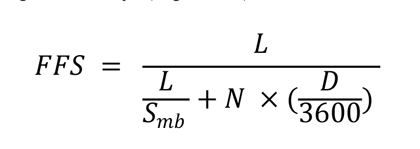Figure 7. Equation. Formula for computing free-flow speed on signalized highways.

Where:

FFS = free-flow speed, accounting for signal delay.
L = length of the facility.
Smb = midblock speed.
N = number of signals.
D = average delay per signal.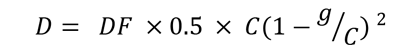Figure 8. Equation. Formula for calculating delay per signal.

Where:

g = effective green time.
C = cycle length.
DF = progression factor.

Defaults:

C = 120 seconds.
g/C = 0.45.
DF = 0.9 for uncoordinated traffic actuated signals.
= 1.0 for uncoordinated fixed time signals.
= 1.2 for coordinated signals with unfavorable progression.
= 0.9 for coordinated signals with favorable progression.
= 0.6 for coordinated signals with highly favorable progression.

The midblock speed, Smb, can be determined using the databased procedure for freeways. Alternately, it can be set to the speed limit.

For PM3 LOTTR percentage of reliable travel, dataset at the subsection level is used given that the calculation entails the aggregation of subsection lengths. It is not available to the trajectory data given the data structure. The calculation process includes:

• Assign the four Federally defined LOTTR periods.
• Calculate the LOTTR values for each subsection in each LOTTR period.
• Determine the reliability of subsections.
• Calculate the ratio of reliable subsections to the facility length.22

For PM3 LOTTR system reliability, facility-level data is grouped by LOTTR periods to determine whether the facility is reliable. This calculation is available to all data sources.23

19 Turner, S., R. Margiotta, and T. Lomax. December 2004. Monitoring Urban Freeways in 2003: Current Conditions and Trends from Archived Operations Data. Report No. FHWA-HOP-05-018. Washington, DC: Federal Highway Administration. [Return to note 19]

21 Transportation Research Board of the National Academies of Sciences, Engineering, and Medicine. 1997. NCHRP Report 387: Planning Techniques to Estimate Speed and Service Volume for Planning Applications. Washington, DC: National Academy of Sciences. [Return to note 21]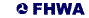United States Department of Transportation - Federal Highway Administration